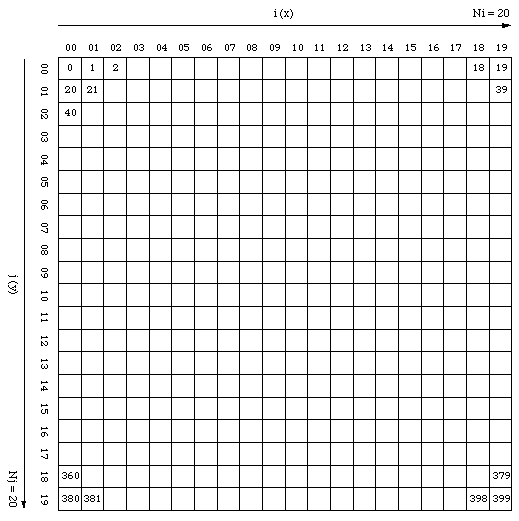# Grid Indexing Convention

(Example for Ni = 20, Nj = 20)

Written by Paul Bourke
November 1997Grid is characterised by the number of cells horizontally Ni and vertically Nj

Grids are saved to files in "cell number" order where

cell number = j * Ni + i

For example
```for (j=0;j<Nj;j++)
for (i=0;i<Ni;i++)
printf("%g",grid[i][j]);
```

Calculate indices (i,j) from the cell number as follows

i = cell number % Ni
j = cell number / Ni